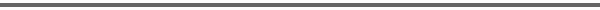Journal Home Page Cumulative Index List of all Volumes Complete Contentsof this Volume Previous Article Next Article Journal of Convex Analysis 10 (2003), No. 2, 295--324 Copyright Heldermann Verlag 2003Relaxation of Variational Functionals with Piecewise Constant Growth Conditions Domenico Mucci Dip. di Matematica, Universitŕ di Parma, Via D'Azeglio 85/A, 43100 Parma, Italy, domenico.mucci@unipr.it[Abstract-pdf] We study the lower semicontinuous envelope of variational functionals given by $\int f(x, Du)\,dx$, for smooth functions $u$, and equal to $+\infty$ elsewhere, under nonstandard growth conditions of $(p,q)$-type: namely, we assume that $$\vert z\vert^{\px}\leq f(x,z)\leq L(1+\vert z\vert^{\px})\,.$$ If the growth exponent is piecewise constant, i.e., $\px\equiv p_i$\, on each set of a smooth partition of the domain, we prove measure and representation property of the relaxed functional. We then extend the previous results by considering $\px$ uniformly continuous on each set of the partition. We finally give an example of energy concentration in the process of relaxation. FullText-pdf (754 K)